# Scientific notation

Scientific notation is a compact form of writing numbers that are very large or very small. It is especially useful when expressing measurements such as distances to stars, eons of time, or the sizes of objects that are extremely large or small. This type of notation helps simplify calculations and comparisons of these numbers, and it helps express the precision with which quantities have been measured.

Each number is expressed in the form of a power of 10. For example, the Earth's mass is about 5,973,600,000,000,000,000,000,000 kilograms (kg). In scientific notation, this number is written as 5.9736×1024 kg. An electron's mass, which is about 0.00000000000000000000000000000091093826 kg, is written as 9.1093826×10−31 kg. The speed of light, which is approximately 300,000,000 meters per second (m/s), is written as 3×108 m/s.

## General form for scientific notation

The general form of writing a number in scientific notation is a×10b, where b is an integer and is called the exponent, and a is any real number, called the significand or mantissa (using "mantissa" may cause confusion because it can also refer to the fractional part of the common logarithm).

In "normalized form," a is chosen such that 1 ≤ a < 10. In other words, a can have any value ranging from 1 to less than 10. (In normalized form, the value of a can be 9.99 but not 10.) Also, the exponent b gives the order of magnitude of the number. The greater the value of b, the larger the overall number; and conversely, the smaller the value of b, the smaller the number. It is assumed that scientific notation should be expressed in normalized form, except during calculations or when an unnormalized form is desired (such as for engineering applications).

## Variations

### Engineering notation

In engineering notation, the exponent b is restricted to multiples of 3. Engineering notation is therefore not always normalized. Numbers in this form are easily read out using magnitude prefixes such as mega or nano. For example, 12.5×10-9 meters might be read or written as "twelve point five nanometers," or 12.5 nm.

### Notation using E

For devices such as calculators, typewriters, and some computers, it may be difficult to show a number with an exponent, such as 107. In such cases, an alternate notation is often used: "×10" is replaced by the letter E or e (for exponent), followed by the exponent. (Note that this e is not related to the mathematical constant e.) The sign of the exponent, whether positive or negative, is often given. For example, 1.56234 E+29 is a representation of the number 1.56234×1029.

## Usefulness

As mentioned above, scientific notation is a convenient way to write large and small numbers and perform calculations with them. In addition, this notation helps avoid misinterpretation of terminology, such as "billion" or "trillion," which have different meanings in different parts of the world. Moreover, scientific notation quickly conveys two properties of a measurement that are useful to scientists: Significant figures and order of magnitude, as explained below.

### Significant digits

Scientific notation is useful for indicating the precision with which a quantity was measured. By including only the significant figures (the digits that are known to be reliable, plus one uncertain digit) in the coefficient, one can convey the precision of the value. In the absence of any statement otherwise, the value of a physical quantity in scientific notation is assumed to have been measured to at least the quoted number of digits of precision, with the last digit potentially in doubt by half a unit.

Consider, for example, the Earth's mass given above in conventional notation (5,973,600,000,000,000,000,000,000 kg). That representation gives no indication of the accuracy of the reported value, so a reader could incorrectly assume from the twenty-five digits shown that the mass is known right down to the last kilogram. By writing the number in scientific notation, one indicates that the Earth's mass is known with a precision of ± 0.00005×1024 kg, or ± 5×1019 kg.

In cases where precision in such measurements is crucial, more sophisticated expressions of measurement error must be used.

### Order of magnitude

Scientific notation also enables simple comparisons of orders of magnitude. For example, a proton's mass, which is 0.0000000000000000000000000016726 kg, can be written as 1.6726×10−27 kg. As noted above, the electron's mass is 9.1093826×10−31 kg. To compare the orders of magnitude of the masses, one can simply compare the exponents rather than counting all the leading zeros. In this case, "−27" is larger than "−31" by the number 4, and therefore the proton is roughly four orders of magnitude (about 10,000 times) more massive than the electron.

## Converting numbers to scientific notation

A number can be readily converted to scientific notation in a few simple steps. Consider the following examples.

Example A

To convert the number 123.4 to scientific notation, one can use the steps given below.

1. Write 123.4 in a form that shows it multiplied by 100. (Note that 100 = 1, so this step is the same as multiplying the number by 1.)$123.4 = 123.4\times10^0$

2. Divide the mantissa (123.4) by 100, by shifting the decimal point two places to the left. Also multiply 100 by 100, by adding 2 to the exponent.$123.4\times10^0 = (123.4/10^2) \times (10^0\times10^2) = 1.234\times10^2$

Thus the scientific notation for 123.4 is 1.234×102.

Example B

To convert the number 0.001234 to scientific notation, one can use the following steps.

1. Write 0.001234 in a form that shows it multiplied by 100.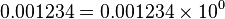$0.001234 = 0.001234\times10^0$

2. Multiply the mantissa (0.001234) by 1,000, by shifting the decimal point three places to the right. Also divide 100 by 1,000, by adding −3 to the exponent.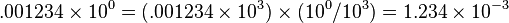$.001234\times10^0 = (.001234\times 10^3) \times (10^0 / 10^3) = 1.234\times10^{-3}$

Thus the scientific notation for 0.001234 is 1.234×10−3.

## Basic operations with numbers in scientific notation

Consider two numbers, x0 and x1, in scientific notation. They may be written as follows.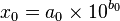$x_0=a_0\times10^{b_0}$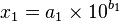$x_1=a_1\times10^{b_1}$

Multiplication and division:

Multiplication and division are performed using the rules for operation with exponential functions: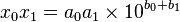$x_0 x_1=a_0 a_1\times10^{b_0+b_1}$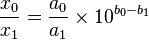$\frac{x_0}{x_1}=\frac{a_0}{a_1}\times10^{b_0-b_1}$

Examples: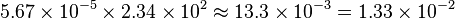$5.67\times10^{-5} \times 2.34\times10^2 \approx 13.3\times10^{-3} = 1.33\times10^{-2}$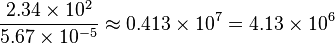$\frac{2.34\times10^2}{5.67\times10^{-5}} \approx 0.413\times10^{7} = 4.13\times10^6$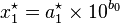$x_1^\star = a_1^\star \times10^{b_0}$$x_0 \pm x_1=x_0 \pm x_1^\star=(a_0\pm a_1^\star)\times10^{b_0}$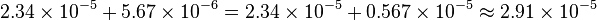$2.34\times10^{-5} + 5.67\times10^{-6} = 2.34\times10^{-5} + 0.567\times10^{-5} \approx 2.91\times10^{-5}$